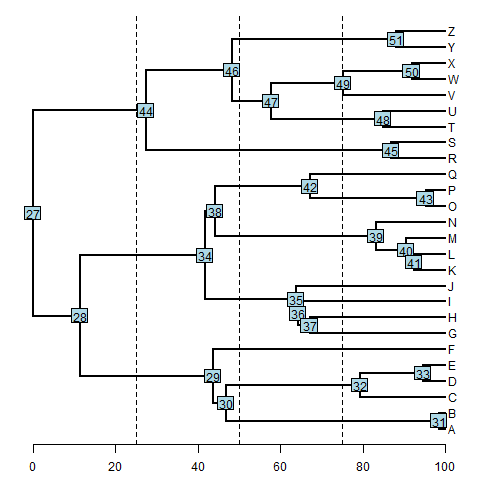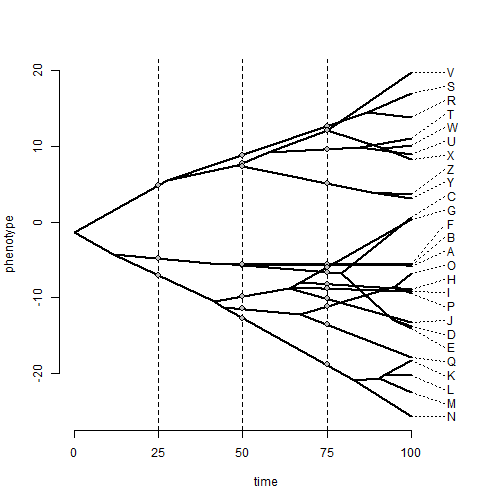## Monday, January 9, 2017

### Extracting reconstructed trait values along edges at different time-slices of a phylogenetic tree

A phytools user contacted me the other day about getting reconstructed internal values instead of at nodes, at different time slices of the tree.

His idea was to extract these from a `"contMap"` object, but since `contMap` is primarily for visualization (and finally discretizes time), a more sensible approach is to simply re-root the tree at each of the desired time slices and compute the values for those slices.

Note that now our values will correspond to points along edges, rather than to number internal nodes.

Here, I'll demo this using a tree with 26 taxa & total depth of 100, sampling edge states at depths 25, 50, & 75 - but roughly the same procedure should work on any tree.

First, here are our data:

``````library(phytools)
tree
``````
``````##
## Phylogenetic tree with 26 tips and 25 internal nodes.
##
## Tip labels:
##  A, B, C, D, E, F, ...
##
## Rooted; includes branch lengths.
``````
``````x
``````
``````##           A           B           C           D           E           F
##  -5.8903745  -5.7282584   0.5445433 -13.8386694 -14.0666611  -5.6192333
##           G           H           I           J           K           L
##   0.2928983  -8.9493757  -9.2341163 -13.2477565 -18.3027044 -20.3522182
##           M           N           O           P           Q           R
## -22.4985786 -25.7573926  -6.8546698  -9.4871527 -17.8719246  13.8470125
##           S           T           U           V           W           X
##  17.0261569  11.0099036   8.9560054  19.7438105  10.0868538   8.2936089
##           Y           Z
##   3.1156954   3.5875638
``````
``````plotTree(tree,mar=c(4.1,1.1,1.1,1.1))
axis(1)
abline(v=c(25,50,75),lty="dashed")
nodelabels()
``````Note that for each time slice, obviously we are going to get different numbers of states (3, 9, & 13 in this case).

Now, let's do it for time slice `t = 25`:

``````H<-nodeHeights(tree)
t<-25
ii<-intersect(which(H[,1]<=t),which(H[,2]>t))
node<-tree\$edge[ii,2]
position<-t-H[ii,1]
rerooted<-mapply(reroot,node=node,position=position,
MoreArgs=list(tree=tree),SIMPLIFY=FALSE)
foo<-function(ind,tree) paste(tree\$edge[ind,],collapse=",")
a25<-setNames(sapply(rerooted,function(t,x) fastAnc(t,x),x=x),
sapply(ii,foo,tree=tree))
a25
``````
``````##     28,29     28,34     27,44
## -4.797286 -7.056366  4.821428
``````

As you can see, I've set the names of `a25` to match the starting & ending node indices as plotted above.

We can of course repeat the same procedure for each of our time slices:

``````## time 50
t<-50
ii<-intersect(which(H[,1]<=t),which(H[,2]>t))
node<-tree\$edge[ii,2]
position<-t-H[ii,1]
rerooted<-mapply(reroot,node=node,position=position,
MoreArgs=list(tree=tree),SIMPLIFY=FALSE)
foo<-function(ind,tree) paste(tree\$edge[ind,],collapse=",")
a50<-setNames(sapply(rerooted,function(t,x) fastAnc(t,x),x=x),
sapply(ii,foo,tree=tree))
a50
``````
``````##      30,31      30,32       29,6      34,35      38,39      38,42
##  -5.685643  -5.788953  -5.558655  -9.855100 -12.692816 -11.479507
##      44,45      46,47      46,51
##   8.854554   7.781511   7.332690
``````
``````## time 75
t<-75
ii<-intersect(which(H[,1]<=t),which(H[,2]>t))
node<-tree\$edge[ii,2]
position<-t-H[ii,1]
rerooted<-mapply(reroot,node=node,position=position,
MoreArgs=list(tree=tree),SIMPLIFY=FALSE)
foo<-function(ind,tree) paste(tree\$edge[ind,],collapse=",")
a75<-setNames(sapply(rerooted,function(t,x) fastAnc(t,x),x=x),
sapply(ii,foo,tree=tree))
a75
``````
``````##      30,31      30,32       29,6       37,7       37,8       36,9
##  -5.748358  -6.702843  -5.588944  -6.015129  -8.228368  -8.797681
##      35,10      38,39      42,43      42,17      44,45      47,48
## -10.128888 -18.896693 -11.157098 -13.541521  12.638761   9.604015
##      47,49      46,51
##  12.091764   5.072981
``````

If we overlay these points on a 'traitgram' style visualization, we should see that they fall directly on the lines of our plot.

``````phenogram(tree,x,spread.cost=c(1,0))
abline(v=c(25,50,75),lty="dashed")
points(rep(25,length(a25)),a25,pch=21,bg="grey",cex=1.2)
points(rep(50,length(a50)),a50,pch=21,bg="grey",cex=1.2)
points(rep(75,length(a75)),a75,pch=21,bg="grey",cex=1.2)
``````You get the idea. Cool!